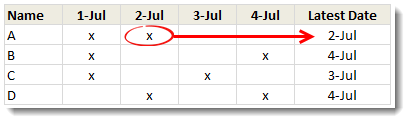# All articles with 'lookup' Tag

## Lookup last non blank value – Excel Challenge

Published on Jun 12, 2020 in Excel Challenges, Learn ExcelI have a fun Excel lookup challenge for you. You have data as shown below and want to find the last non blank value for a given account number. For example, for acct number 2015, the answer would be Freedom. How would you solve this? Refer to this workbook for 3 possible answers. Just move […]

Continue »

## Figure out slot from given time [quick tip]

Published on Apr 19, 2016 in Excel Howtos, Quick TipHere is an interesting scenario.

Let’s say you are looking at a time, like 9:42 AM and want to know which 15 minute slot it fits into. The answer is 9:30 – 9:45. But how would you get this answer thru Excel formulas?

Continue »

## CP002: VTALKUP – 5 Excel lookup functions demystified + 4 Resources for you

Published on Mar 13, 2014 in Chandoo.org Podcast SessionsIn the second session of Chandoo.org Podcast, We will be learning how to use 5 Excel lookup functions.

What is in this session?
In this session, we tackle one of the most important areas of Excel. The lookup functions.

You will learn,

• Why lookup functions are necessary
• 5 Important lookup functions in Excel – VLOOKUP, HLOOKUP, LOOKUP, MATCH & INDEX
• When & how to use each of these 5 functions?
• Extreme scenarios:
• What happens when the value you are looking up is not there?
• What if too many items match the lookup value?
• What if you have too many conditions in the lookup criteria?
• Using IFERROR function
• Re-cap of the new powers you acquired
• 4 Resources for you to learn lookup functions better
Continue »

## Formula Forensics-No. 036: Calculating Costs that Vary by Year and Age

Published on Nov 26, 2013 in Excel Howtos, Formula Forensics, Posts by Sajan

In this episode of Formula Forensics, lets talk about how to build a model where costs vary per year and age. In this example, we are talking about a plantation project. The costs & yields of plants vary by the year they are planted in and the age of plants. In this case, how do we calculate the total costs or returns with formulas?Continue »

## Formula Forensics No. 030 – Extracting a Sorted, Unique List, Grouped by Frequency of Occurrence

Published on Oct 4, 2012 in Formula Forensics, Posts by SajanToday at Formula Forensics, Guest author Sajan shows us how to extract a sorted, unique list of items, displaying the most frequently occurring items first, while restricting the output based on some additional criteria.

Continue »

## Find the last date of an activity

Published on Jul 3, 2012 in Formula Forensics, Learn ExcelWe know that using VLOOKUP, we can find a value corresponding to a given item. For example Sales of x. But what if you have multiple sales for each item and you want the last value?

Today lets understand how to find the last date of an activity, given data like above.

Like everything else in Excel, there are multiple ways to finding last date. If cats can use computers, they would hate Excel. You see, Excel is overflowing with unlimited ways to skin a cat.

Continue »

### Get FREE Excel & Power-BI Newsletter

One email per week with Excel and Power BI goodness. Join 100,000+ others and get it free.